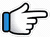# Aapki Nazron Ne Samjha – Keyboard Notes## Aapki Nazron Ne Samjha Keyboard NotesFor Aapki Nazro Ne Samjha Notes as Western or Indian Notations, Click –

Western –
Indian –

For Full Song Lyrics

## Keyboard Notes for Aapki Nazro Ne Samjha – Indian Notation (srg mpdn)

R*nn      n        R*dn         n      dMM
Aa~p      ki      nazron     ne     samjha
Or
R*~ n    n        R*dn        R*    dMM

r*~        r*g*~   R*nnd      dR*r*R*ndpdnR*
pyar      ke        kaabil       mujhe~~~
Or
r*~ r*    g*r*   R*nnd     dr*R*ndp

R*n  n    n~     R*dn             ndM        M
Dil    ki   ae    dhadakan     thahar     ja

r*~       r*g*~     R*nnd       dR*r*R*ndpdn
mil      gayee     manjil       mujhe
Or
r*~     r*g*r*    R*nnd     dr*R*ndp

r*g*R*n~~     R*dn        n      n~ n
Aapki             nazron     ne     samjha

Music:
R*~        r*g*R*
nnMn~    R*r*n
MMgd   Dnd
MrMpd  DR*nR*dn DdMn

n~      dn           r*R*nd~ p      Mpdn
Jee    hamen    manzoo~~r       hai~~,

d~p Mr      rg     d~ pM r* R* ndn
aap  ka      ye     faisalaa~~~
Or
d~~p M~   rr     d~ pM r* R* ndn

n~      dn           r*R*nd~ p      Mpdn
Jee    hamen    manzoo~~r       hai~~,

d~~p Mr    rg     d~ pdn~
aap  ka      ye     faisalaa~~~

R*~     R*R*       R*     r*~     R*r*R*ndp
Kah     rahee    hai     har     nazar

p~ d          R*g*r*d*       n~ n dndn pd
banda      paravar        shukriya~~~~
Or
p~ d         pdR*n          n~ ndnR*ndp

R*n     n       n~ R*d       n~ R*dM      M
Hans_ ke     apanee      zindagi         mein
Or
R*n     n        n~ R*d        n~ ndp         M

r*~     r*g*      R*nnd       dR*r*R*ndpdnR*
kar     liya       shaamil     mujhe
Or
r*~ r*    g*r*   R*nnd     dr*R*ndp

R*n  n    n~     R*dn             ndM        M
Dil    ki   ae    dhadakan     thahar     ja

r*~       r*g*~     R*nnd       dR*r*R*ndpdn
mil      gayee     manjil       mujhe
Or
r*~     r*g*r*    R*nnd     dr*R*ndp

r*g*R*n~~     R*dn        n      n~ n
Aapki             nazron     ne     samjha

Starting Music:
Mpd Mpd    R*n~~
nR*g*M*p*d*n*
*ngdpMn

Will be Updated:
Aap ki manzil hoon main,
meree manzil aap hain
Kyon main toofaa se daroo
mera saahil aap hain
Koyee toofaano se kah de
mil gaya saahil mujhe
Dil kee ae dhadakan thahar ja
mil gayee manjil mujhe
Aapki nazron ne samjha